#Learning Data Science and Machine Learning: First Steps After The Roadmap

Just getting into learning data science may seem as daunting as (if not more than) trying to land your first job in the field. With so many options and resources online and in traditional academia to consider, these pre-requisites and pre-work are recommended before diving deep into data science and AI/ML.Source: https://www.wiplane.com/p/foundations-for-data-science-ml

At the start of this year, I published a mind map on the Data Science learning roadmap (shown below). The roadmap was widely accepted, that article got translated into different languages, and a large number of folks thanked me for publishing it.

Everything was good until a few aspirants pointed out that there are too many resources and many of them are expensive. Python programming was the only branch that had a number of really good courses, but it ends right there for beginners.

A few important questions on foundational data science struck me:

• What should one do after learning how to code? Are there topics that help you strengthen your foundations for data science?
• I hate math, and there are either very basic tutorials or too deep for me. Can you recommend a compact yet comprehensive course on Math and Statistics?
• How much math is enough to start learning how ML algorithms work?
• What are some essential statistics topics to get started with data analysis or data science?

Answers to a lot of these questions can be found in the book Deep Learning by Ian Goodfellow and Yoshua Bengio.But that book is a bit too technical and math-heavy for many.

So here goes the essence of this article, the first steps to learning data science or ML.

## The Three Pillars of Data Science & ML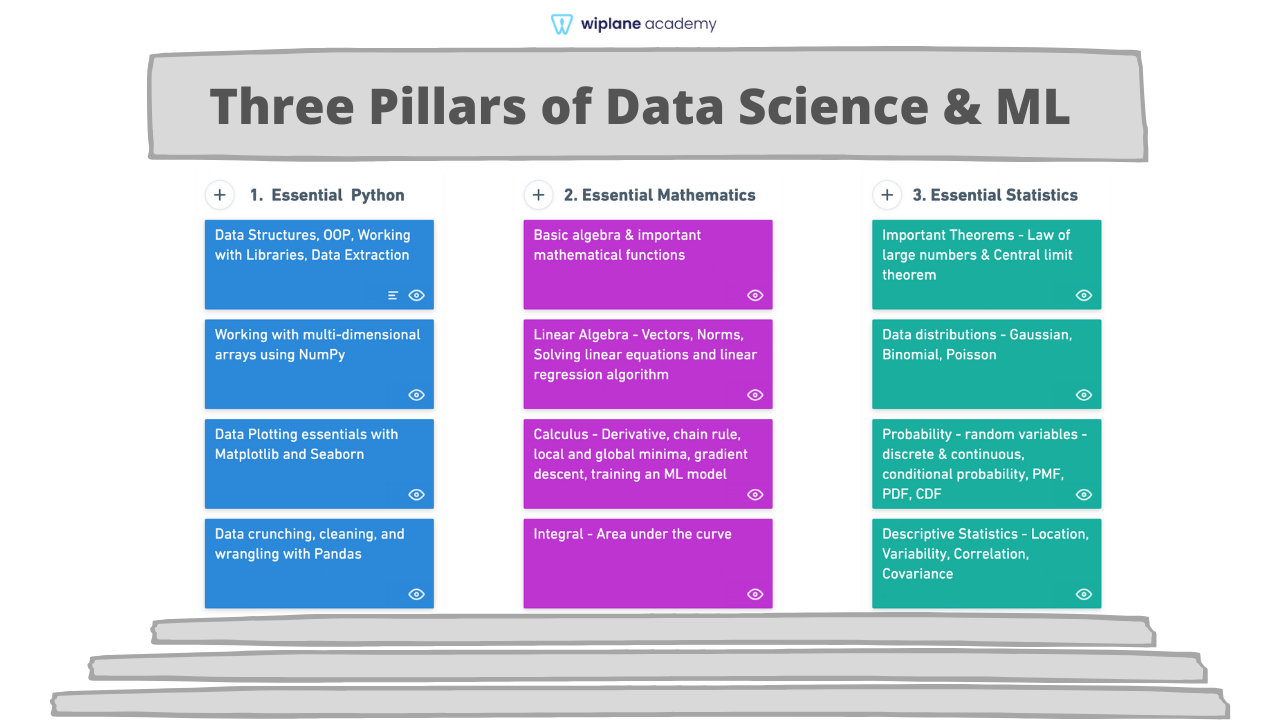Source: https://wiplane.com

If you go through the pre-requisites or pre-work of any ML/DS course, you’ll find a combination of programming, math, and statistics.

Forgetting about the others for now, here is what Google recommends that you do before doing an ML course: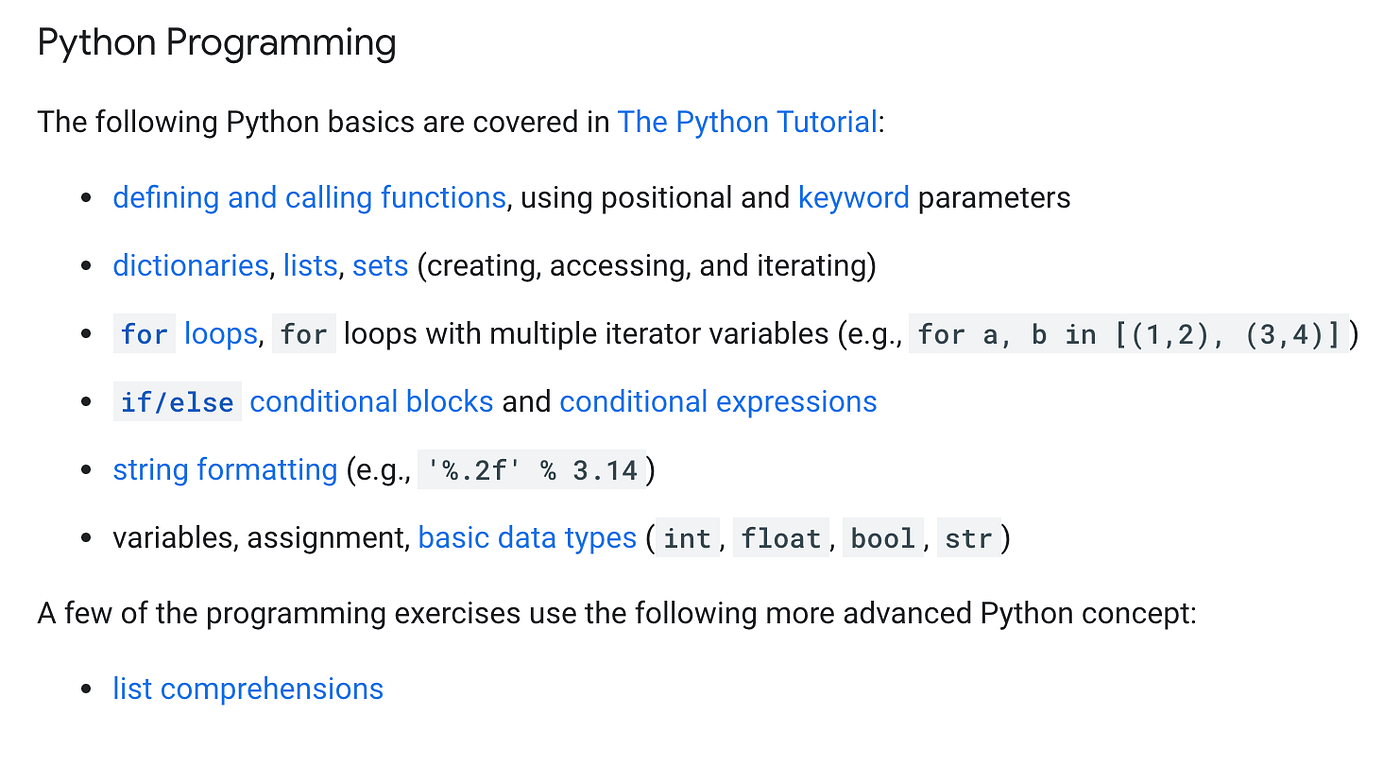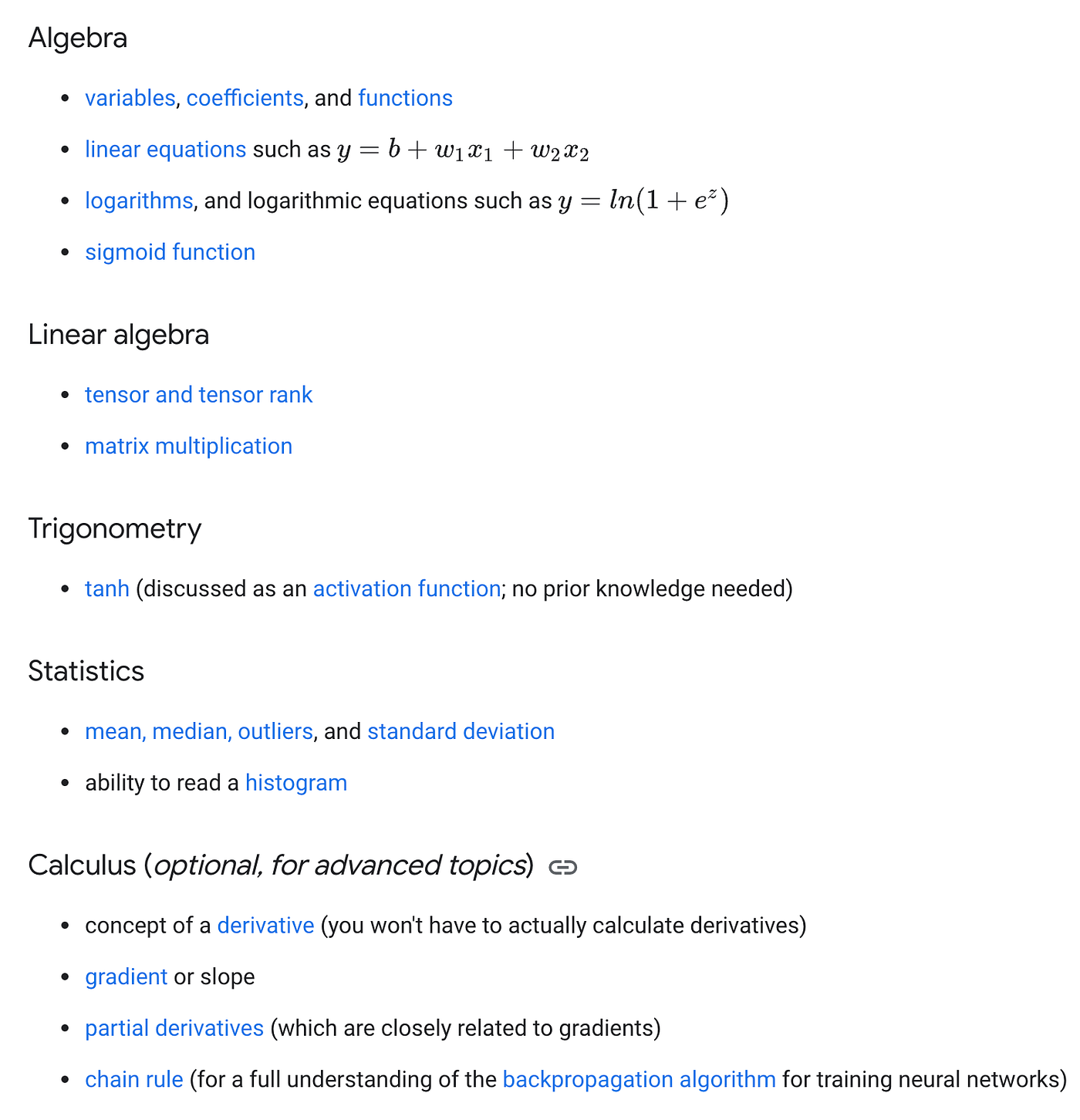### 1. Essential Programming

Most data roles are programming-based except for a few like business intelligence, market analysis, product analyst, etc.

I am going to focus on technical data jobs that require expertise in at least one programming language. I personally prefer Python over any other language because of its versatility and ease of learning—hands-down, a good pick for developing end-to-end projects.

A glimpse of topics/libraries one must master for data science:

• Common data structures (data types, lists, dictionaries, sets, tuples), writing functions, logic, control flow, searching and sorting algorithms, object-oriented programming, and working with external libraries.
• Writing python scripts to extract, format, and store data into files or back to databases.
• Handling multi-dimensional arrays, indexing, slicing, transposing, broadcasting and pseudorandom number generation using NumPy.
• Performing vectorized operations using scientific computing libraries like NumPy.
• Manipulate data with Pandas— series, dataframe, indexing in a dataframe, comparison operators, merging dataframes, mapping, and applying functions.
• Wrangling data using Pandas— checking for null values, imputing it, grouping data, describing it, performing exploratory analysis, etc.
• Data Visualization using Matplotlib— the API hierarchy, adding styles, color, and markers to a plot, knowledge of various plots and when to use them, line plots, bar plots, scatter plots, histograms, boxplots, and seaborn for more advanced plotting.

### 2. Essential Mathematics

There are practical reasons why math is essential for folks who want a career as an ML practitioner, Data Scientist, or Deep Learning Engineer.

#1 Linear algebra to represent data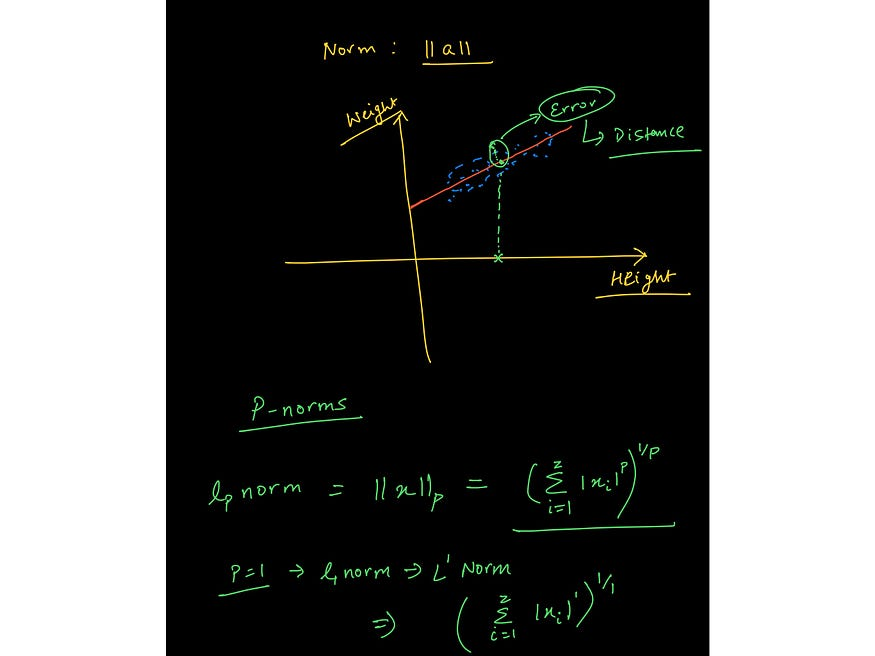An image from the lecture on Vector Norms from the course: https://www.wiplane.com/p/foundations-for-data-science-ml

ML is inherently data-driven because data is at the heart of machine learning. We can think of data as vectors — an object that adheres to arithmetic rules. This leads us to understand how rules of linear algebra operate over arrays of data.

#2 Calculus to train ML models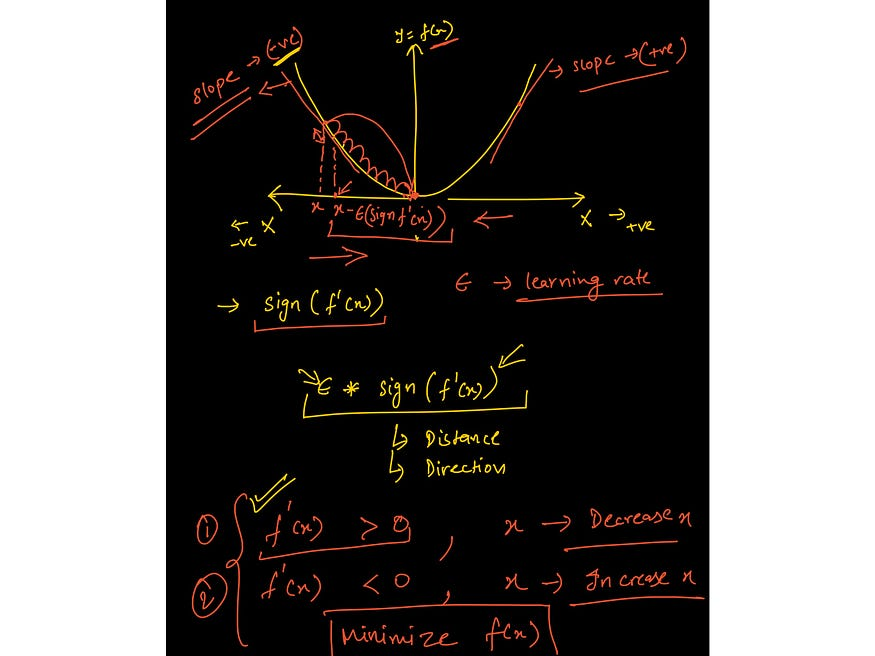An image from the lecture on gradient descent from the course: https://www.wiplane.com/p/foundations-for-data-science-ml

If you are under the impression that a model training happens “automatically,” then you are wrong. Calculus is what drives the learning of most ML and DL algorithms.

One of the most commonly used optimization algorithms—gradient descent—is an application of partial derivatives.

A model is a mathematical representation of certain beliefs and assumptions. It is said to learn (approximate) the process (linear, polynomial, etc.) of how the data is provided, was generated in the first place, and then make predictions based on that learned process.

Important topics include:

• Basic algebra — variables, coefficients, equations, and linear, exponential, logarithmic functions, etc.
• Linear Algebra — scalars, vectors, tensors, Norms (L1 & L2), dot product, types of matrices, linear transformation, representing linear equations in matrix notation, solving linear regression problem using vectors and matrices.
• Calculus — derivatives and limits, derivative rules, chain rule (for backpropagation algorithm), partial derivatives (to compute gradients), the convexity of functions, local/global minima, the math behind a regression model, applied math for training a model from scratch.

#3 Essential Statistics

Every organisation today is striving to become data-driven. To achieve that, Analysts and Scientists are required to use put data to use in different ways in order to drive decision making.

Describing data — from data to insights

Data always comes in raw and ugly. The initial exploration tells you what’s missing, how the data is distributed, and what’s the best way to clean it to meet the end goal.

In order to answer the defined questions, descriptive statistics enables you to transform each observation in your data into insights that make sense.

Quantifying uncertainty

Furthermore, the ability to quantify uncertainty is the most valuable skill that is highly regarded at any data company. Knowing the chances of success in any experiment/decision is very crucial for all businesses.

Here are a few of the main staples of statistics that constitute the bare minimum: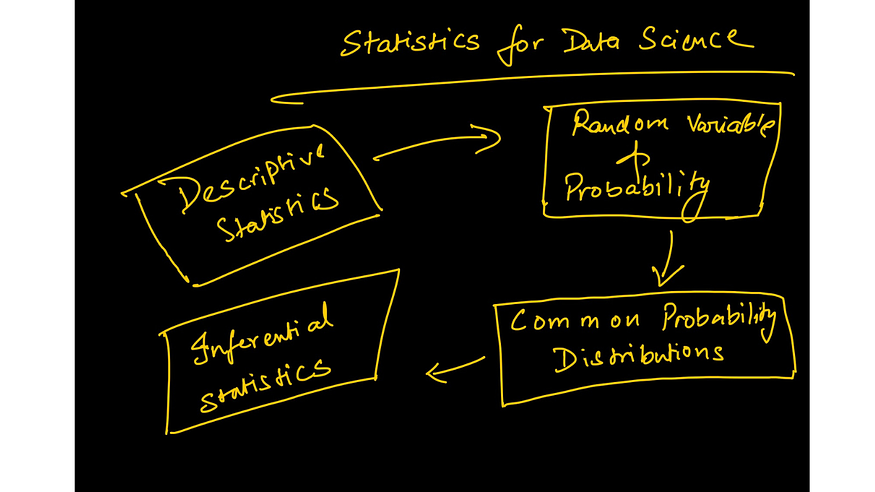Image from the lecture on Poisson distribution — https://www.wiplane.com/p/foundations-for-data-science-ml

• Estimates of location — mean, median, and other variants of these.
• Estimates of variability
• Correlation and covariance
• Random variables — discrete and continuous
• Data distributions— PMF, PDF, CDF
• Conditional probability — Bayesian statistics
• Commonly used statistical distributions — Gaussian, Binomial, Poisson, Exponential
• Important theorems — Law of large numbers and Central limit theorem.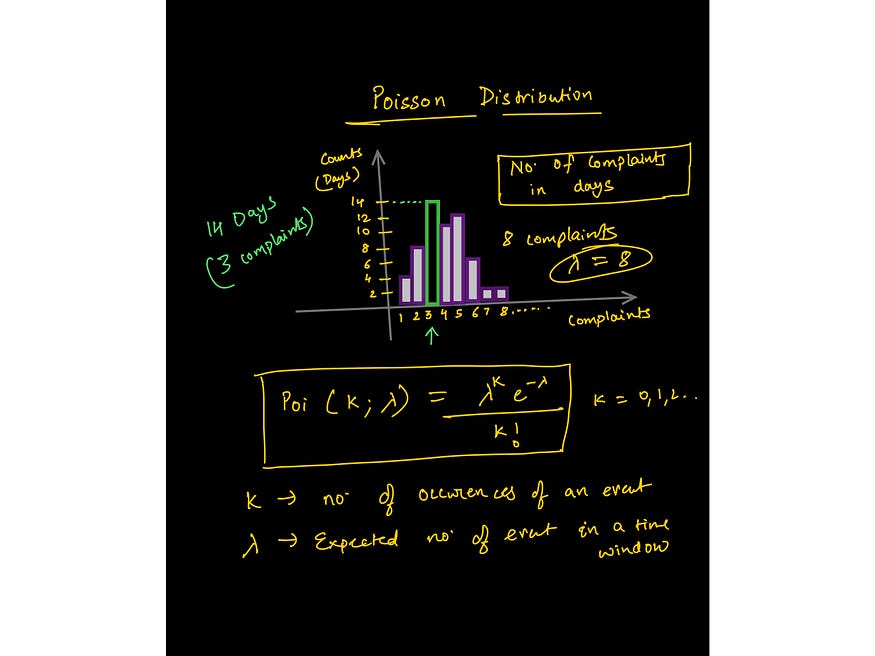Image from the lecture on Poisson distribution — https://www.wiplane.com/p/foundations-for-data-science-ml

• Inferential Statistics — A more practical and advanced branch of statistics that helps in designing hypothesis testing experiments, pushes us to understand the meaning of metrics deeply and at the same time helps us in quantifying the significance of the results.
• Important tests — Student’s t-Test, Chi-Square test, ANOVA test, etc.

Every beginner-level data science enthusiast should focus on these three pillars before diving into any core data science or core ML course

## Resources to learn the above — in search of a compact, comprehensive yet affordable course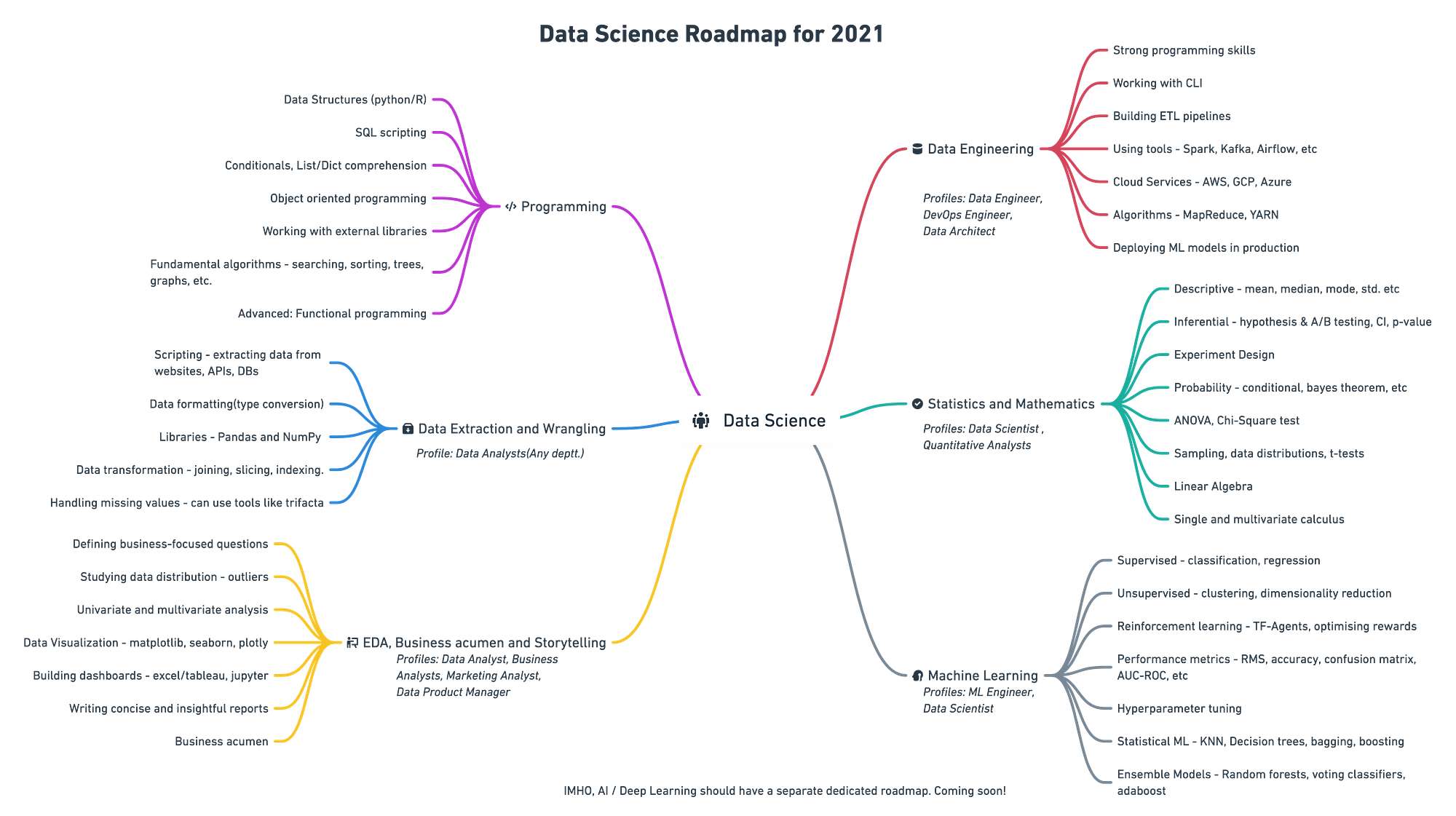My learning roadmap also told you what to learn, and it was also loaded with resources, courses, and programs that one can enroll themselves in.

But there are a few inconsistencies in the recommended resources and the roadmap that I had charted out.

### Problems with Data Science or ML Courses

1. Every data science course that I enlisted there required students to have a decent understanding of programming, math, or statistics. For example, the most famous course on ML by Andrew Ng also relies heavily on the understanding of vector algebra and calculus.
2. Most courses that cover math and statistics for Data Science are just a checklist of concepts required for DS/ML with no explanation on how they are applied and how they are programmed into a machine.
3. There are exceptional resources to dive deep into math, but most of us are not made for it, and one doesn’t need to be a gold medalist to learn data science.

Bottom line: A resource that covers just enough applied math or statistics or programming to get started with data science or ML is missing.

So, I decided to give in and do it all myself. I have spent the last 3 months developing a curriculum that will provide a solid foundation for your career as a…

• Data Analyst
• Data Scientist
• Or an ML Practitioner/Engineer

Here I present you the Foundations for Data Science or ML — First Steps to learn Data Science and MLThat’s me when I decided to launch!

A comprehensive yet compact and affordable course that not only covers all the essentials, pre-requisites, and pre-work but also explains how each concept is used computationally and programmatically (Python).

And that’s not all. I will keep updating the course content every month based on your input. Learn more here.

Early Bird Offer!

I am stoked to launch the pre-sales of this course as I am currently in the process of recording and editing the final bits of 2–3 modules, which will also be live by the first week of September.

Grab the early bird offer, which is only valid until August 30, 2021.

Original. Reposted with permission.

Bio: Harshit Tyagi is an engineer with amalgamated experience in web technologies and data science (aka full-stack data science) who has mentored over 1000 AI/Web/Data Science aspirants, while designing data science and ML engineering learning tracks. Previously, Harshit developed data processing algorithms with research scientists at Yale, MIT, and UCLA.

Related: